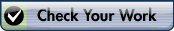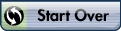﻿ Exam review

Name (not needed):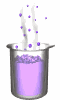Chemistry - Final Exam ReviewTrue/False
Indicate whether the statement is true or false.

1.

All samples of the same substance have the same density.

2.

Objects with a density greater than water will sink in water.

3.

Density can be expressed in g/ml or g/cm3.

4.

Absolute Zero, 0K = 273°C

5.

Compounds are two or more substances which are not chemically comined

6.

The following graph shows a DIRECT relationship between Volume and Pressure.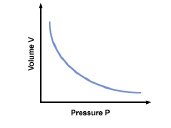7.

Increasing the number of particles, heating, or decreasing volume will all cause an increase in pressure.

8.

An isotope is an element with a positive or negative charge.

9.

the resulting energy from electrons moving from lower to higher energy levels is what causes the colored light that is produced in flame tests and neon lights.

10.

The modern periodic table (the one that we use now) is arranged in order of increasing atomic number.

11.

Non-metals are located in the upper right hand side of the periodic table.

12.

Ionic bonds are formed by the sharing of electrons.

13.

In a chemical reaction, the products are found on the left side of the arrow.

14.

A precipitate forms when two aqueous solutions react to form a product that cannot dissolve in water.

15.

Something that is insoluble will NOT dissolve in water

16.

The solute is what dissolves the solvent in a solution.

17.

A pH value of 10 is considered to be neutral.

Matching

Match the energy level with the maximum number of electrons that it can hold.
 a. 2 b. 8 c. 18

18.

the third evergy level

19.

the first energy level

20.

the second energy level

Multiple Choice
Identify the choice that best completes the statement or answers the question.

21.

Which of the following indicates a quantitative measurement?
 a. cold c. big b. 55.0 g d. yellow

22.

Which of the following is a physical property?
 a. Melting point c. color b. density d. all of the above

23.

Which is the correct measurement for location marked by the arrow?a. 60.25 c. 62.7 b. 62 d. 62.659

24.

What is the measurement 0.000 024 cm in proper scientific notation?
 a. 2.40 x 10-5cm. c. 240 x 10-4cm. b. 2.40 x 10-4 cm. d. 2.40 x 10-6cm.

25.

An object has a mass of 18.5 kg. What is the mass of the object in grams? (1000 g = 1 kg)
 a. 1.85 x 104 g c. 185 x 10-1 g b. 18.5 x 103 g d. 1.85 x 10-4 g

26.

What is the volume of the following rock?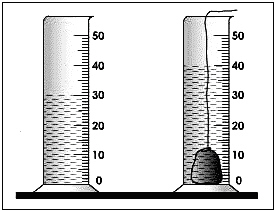a. 30 mL c. 70 mL b. 40 mL d. 10 mL

27.

A group of students performed an experiment to calculate the mass of a substance. The accepted value for the mass should be 2.85 grams. The group recorded the following measurements after performing the experiment three times. Which statement correctly describes the experimental data?
 Trial Measurement 1 2.01 2 3.05 3 2.75
 a. Precise but not accurate c. Accurate but not precise b. Both accurate and precise d. Neither accurate nor precise

28.

Silver, Copper and Oxygen are all:
 a. elements c. mixtures b. compounds d. metals

29.

Which of the following is NOT a state of matter
 a. solid c. cold b. gas d. liquid

30.

According to the Kinetic Molecular Theory, which of the following is not in motion?
 a. solids c. gases b. liquids d. none of these are correct.

31.

Which of the following states of matter has the MOST amount of energy?
 a. solids c. gases b. liquids d. none of these have energy.

32.

The process by which particles gain enough energy at the surface of a  liquid to become a gas is known as?
 a. Evaporation c. Freezing b. Deposition d. Sublimation

33.

Between which two points does thermal energy increase and boiling occur for water?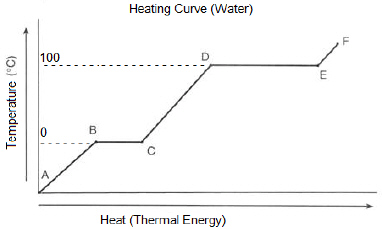a. A to B c. C to D b. B to C d. D to E

34.

Accoriding to Boyle’s law, when volume decreases at a constant temperature the following will happen:
 a. pressure stays the same c. pressure increases b. pressure decreases d. none of the above

35.

According to Bohr’s model of the atom, electrons must:
 a. not exist. b. be anywhere they want outside of the nucleus. c. reside in the nucleus. d. move in “orbits” around the nucleus like planets.

36.

Which of the following are found inside the nucleus?
 a. protons b. neutrons c. electrons d. a and b e. a and c

37.

The number of protons in an atom is always the same as it’s
 a. mass number c. isotope number b. atomic number d. nuetrons

38.

Atoms are neutral when:
 a. protons and neutrons are equal c. protons and electrons are equal b. neutrons and electrons are equal d. none of these are correct.

39.

24. Which of the following combinations describes an ion with a positive two charge?
 a. 1 protons; 0 neutrons; 2 electrons c. 20 protons; 20 neutrons; 18 electrons b. 15 protons; 16 neutrons; 15 electrons d. 11 protons; 12 neutrons; 10 electrons

40.

An atom’s mass number is the total of the:
 a. protons and neutrons c. protons and electrons b. neutrons and electrons d. protons, neutrons and electrons

41.

Two neutral isotopes of the same element have a different number of:
 a. protons b. neutrons c. electrons d. a and b e. a and c

42.

Which pair of symbols correctly represents two isotopes of the same element?
 a.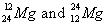c.b.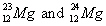d. none of these represent isotopes.

43.

Which is the net charge on a particle that consists of 11 protons, 12 neutrons, and 10 electrons?
 a. 1+ c. 2+ b. 1- d. 2-

44.

Which is the mass number on a particle that consists of 11 protons, 12 neutrons, and 10 electrons?
 a. 21 c. 23 b. 22 d. 33

45.

Which is the number of electrons in the Mg2+ ion?
 a. 24.3 c. 11 b. 12 d. 10

46.

Lithium has an atomic mass of 6.9. which is the best explanation for this?
 a. all lithium atoms have 6.9 mass units in their nuclei. b. The weighted average of all lithium isotopes is 6.9. c. all lithium atoms have a mass of 6.9.

47.

The amount of time that it takes for 1/2 of the mass of a radioactive isotope to decay is known as a radioisotope’s:
 a. decay time c. half time b. radioactive time d. half life

48.

The strongest type of radiation is known as:
 a. beta radiation c. alpha radiation b. gamma radiation d. delta radiation

49.

Complete the following alha decay of Uranium-238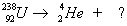a.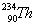b.c.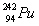d.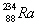50.

Dimitri Mendeleev was known for his work on:
 a. I’ve never heard of him. c. the discovery of the proton. b. his model of the atom d. the first periodic table.

51.

Which of the following elements is most similar in properties to Nitrogen, N?
 a. O b. P c. Ne d. Si

52.

The energy level of the valence shell ist he same as the ________ that an element can be found in.
 a. period number c. atomic number b. mass number d. group number

53.

Element number 37 is a(n):
 a. halogen c. alkali metal b. transition metal d. alkaline earth metal

54.

Which of the following is a transition metal?
 a. Co b. C c. U d. Ne

55.

Which of the following elements are BOTH noble gases?
 a. N and Ne c. Ne and Xe b. Cl and F d. H and He

56.

From the top of the column 1 to the bottom of column 1 on the periodic table:
 a. atomic radius decreases c. reactivity decreases b. atomic mass decreases d. atomic radius increases

57.

Which element will have the same number of electrons as Ar when it forms a bond?
 a. Ne b. K c. F d. Mg

58.

Which of the following is the correct number of valence electrons for Ca?
 a. 1 b. 2 c. 3 d. 4

59.

Which of the following has a full set of valence electrons?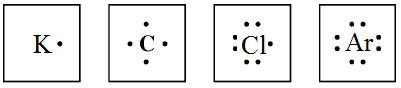a. K c. Cl b. C d. Ar

60.

How does K achieve a stable octet?
 a. it gains 1 electron c. it loses 1 electron b. it gains 7 electrons d. it does not do anything

61.

w does an ionically bonded compound such as, potassium chloride (KCl) formed?
 a. A potassium atom loses an electron to chlorine to form an ionic bond. b. a chlorine atom loses seven electrons to form a cation and potassium atom gains seven electrons to form an ionic bond. c. potassium and chlorine do not form a bond.

62.

Which of the following is true for ionic compounds?
 a. high melting and boiling points c. usually solid at room temperature b. conduct electricity in water d. all of the above

63.

In an ionic compound of potassium oxide, the potassium ion has a charge of 1+ and the oxide ion has a charge of 2-. Which of the following is the formula for potassium oxide?
 a. K2O b. KO2 c. K2O2 d. 2KO

64.

A structural formula with a triple covalent bond will have how many dashes between two atoms?
 a. 1 c. 3 b. 2 d. 0

65.

What is the name of LiF?
 a. lithium fluoride c. lithium monofluoride b. monolithium monofluoride d. Monolithium fluoride

66.

The type of formula that uses dashes to represent bonds is known as what type of formula?
Example: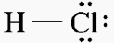a. math formula c. dash formula b. structure formula d. dot formula

67.

How many oxygens are shown in the formula?:    Mg3(PO4)2
 a. 2 b. 4 c. 6 d. 8

68.

What is the molar mass of NaOH?
 a. 40 g b. 20 g c. 23 g d. 32 g

69.

Complete the following statement of equality:
1 mol N = _?_ grams N = 6.02 x 1023 N atoms
 a. 14 b. 7 c. 0 d. none of these

70.

What is the mass of 2 moles of He atoms?
 a. 2 g c. 8 g b. 4 g d. 1.20 x 1024 g

71.

Which of the following has the greatest mass?
 a. 1 mol of Ag c. 1 mol of Au b. 1 mol of Al d. 1 mol of Ar

72.

The percentage composition of carbon in CH4 is about 75%. What ist he percentage of hydrogen in this compound?
 a. 75% b. 25% c. 100% d. 12%

73.

The approximate mass of one silicon atom is about equal to:
 a. one half the mass of one nitrogen molecule (N2) b. the mass of one nitrogen atom (N) c. the mass of one nitrogen molecule (N2)

74.

To calculate the Molarity of a solution, you mus calculate:
 a. liters per grams. c. moles per liters. b. grams per liters. d. iters per moles.

75.

According to the law of conservation of mass, the total mass of the reactants are
 a. always more than the total mass of the products. b. always less than the total mass of the products. c. sometimes more and sometimes less than the total mass of the products. d. always equal to the total mass of the products.

76.

In this equation, which are the reactant(s)?a. Mg only c. Mg + 02 b. Mg0 only d. 02 only

77.

Which is the name of the kind of solid substance formed in this figure?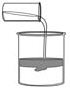a. aqueous c. coordinate complex b. precipitate d. synthesis

78.

In the reaction represented by the equation N2 + 3H2 ® 2NH3, what is the mole ratio of hydrogen to ammonia?
 a. 1:1 b. 2:1 c. 3:2 d. 6:8

79.

For the reaction represented by the equation C + 2H2 ® CH4,
how many moles of hydrogen are required to produce 4 mol of methane, CH4?
 a. 3 mol b. 4 mol c. 8 mol d. 1 mol

80.

Which describes the function of a catalyst?
 a. It speeds up a reaction. c. It makes a reaction exothermic. b. It slows down a reaction. d. It makes a reaction endothermic.

81.

The coefficients in a chemical equation represent the
 a. masses, in grams, of all reactants and products. b. relative numbers of moles of reactants and products. c. number of atoms in each compound in a reaction. d. number of valence electrons involved in the reaction.

82.

What type of chemical reaction releases energy to it’s surroundings?
 a. endothermic b. exothermic c. both endothermic and exothermic d. neither endothermic nor exothermic

83.

Which type of chemical reaction is shown?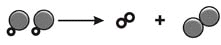a. double replacement c. synthesis b. combustion d. decomposition

84.

Which type of reaction is Al  +  O2  ® Al2O3?
 a. synthesis c. single replacement b. decomposition d. combustion

85.

What are the correct coefficients when this equation is balanced?
____K  +  ____Br2 ®  ____KBr
 a. 1, 1, 1 c. 2, 1, 2 b. 1, 2, 1 d. 2, 1, 1

86.

Which type of chemical reaction would this be classified as always producing carbon dioxide and water like the following:
C3H8  +  O2  ®  CO2  + H2O
 a. synthesis c. combustion b. double replacement d. decomposition

87.

How many grams of hydrogen will be required to produce 32.0 grams of methane from reacting 24 grams of carbon?       given:    Hint: plug in the numbers
C + 2H2 ® CH4
 a. 8 b. 12 c. 56 d. 24

88.

What mass of methane is produced when 36.0 grams of carbon react with 12 grams of hydrogen?
given:
C + 2H2 ® CH4
 a. 8 b. 12 c. 16 d. 48

89.

When an acid is mixed with a base, __________________ occurs.
 a. a physical change c. neutralization b. acidification d. nothing

90.

The following can be indications of an exothermic chemical change during a reaction where NO HEAT IS ADDED.
 a. bubbles and gas form c. both of these b. heat is produced d. neither of these